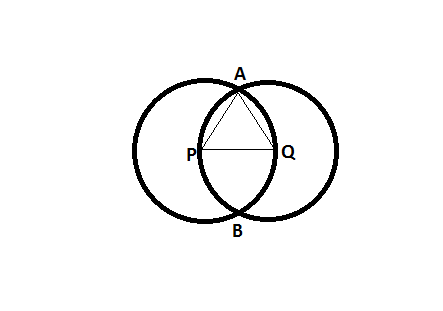Question 24

# Two circles with centres P and Q cut each other at two distinct points A and B. The circles have the same radii and neither P nor Q falls within the intersection of the circles. What is the smallest range that includes all possible values of the angle AQP in degrees?

SolutionTo know the range, we have to take the limiting case.

The limiting case in this case is when the circles pass through each other's centers.

In this case, PQ = AP = AQ => They form an equilateral triangle => angle AQP = 60 degrees.

So, the maximum possible angle is 60 degrees.

Another limiting case is when the circles touch each other externally.

In this case, angle AQP = 0 degrees.

Hence, the range is 0 to 60.